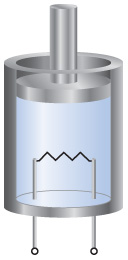# Problem: A gas is confined to a cylinder fitted with a piston and an electrical heater, as shown here:Suppose that current is supplied to the heater so that 100 J of energy is added. Consider two different situations. In case (1) the piston is allowed to move as the energy is added. In case (2) the piston is fixed so that it cannot move.Identify the sign (positive, negative, or zero) of q and w in case (1).

###### FREE Expert Solution

Internal Energy:

$\overline{){\mathbf{∆}}{\mathbf{E}}{\mathbf{=}}{\mathbf{q}}{\mathbf{+}}{\mathbf{w}}}$

+ q → if the system gains, takes in, or absorbs heat or energy
– q → if the system loses, evolves, gives off, or releases heat or energy

90% (181 ratings)###### Problem Details

A gas is confined to a cylinder fitted with a piston and an electrical heater, as shown here:Suppose that current is supplied to the heater so that 100 J of energy is added. Consider two different situations. In case (1) the piston is allowed to move as the energy is added. In case (2) the piston is fixed so that it cannot move.

Identify the sign (positive, negative, or zero) of q and w in case (1).